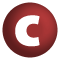# MessageQueue

#### class MessageQueue extends MQueue

The class `MessageQueue` provides an efficient implementation of a message queue specialized for this actor library. Classes in this package are supposed to be the only clients of this class.

1. MQueue
2. AnyRef
3. Any

### Value Members

2. #### def equals(arg0: Any): Boolean

This method is used to compare the receiver object (`this`) with the argument object (`arg0`) for equivalence

This method is used to compare the receiver object (`this`) with the argument object (`arg0`) for equivalence.

The default implementations of this method is an equivalence relation:

• It is reflexive: for any instance `x` of type `Any`, `x.equals(x)` should return `true`.
• It is symmetric: for any instances `x` and `y` of type `Any`, `x.equals(y)` should return `true` if and only if `y.equals(x)` returns `true`.
• It is transitive: for any instances `x`, `y`, and `z` of type `AnyRef` if `x.equals(y)` returns `true` and `y.equals(z)` returns `true`, then `x.equals(z)` should return `true`.

If you override this method, you should verify that your implementation remains an equivalence relation. Additionally, when overriding this method it is often necessary to override `hashCode` to ensure that objects that are "equal" (`o1.equals(o2)` returns `true`) hash to the same `Int` (`o1.hashCode.equals(o2.hashCode)`).

arg0

the object to compare against this object for equality.

returns

`true` if the receiver object is equivalent to the argument; `false` otherwise.

definition classes: AnyRef ⇐ Any
3. #### def extractFirst(p: (Any, OutputChannel[Any]) ⇒ Boolean): MQueueElement

Extracts the first message that satisfies the predicate `p` or `null` if `p` fails for all of them

Extracts the first message that satisfies the predicate `p` or `null` if `p` fails for all of them.

definition classes: MQueue

6. #### def get(n: Int)(p: (Any) ⇒ Boolean): Option[Any]

Returns the n-th message that satisfies the predicate `p` without removing it

Returns the n-th message that satisfies the predicate `p` without removing it.

definition classes: MQueue
7. #### def hashCode(): Int

Returns a hash code value for the object

Returns a hash code value for the object.

The default hashing algorithm is platform dependent.

Note that it is allowed for two objects to have identical hash codes (`o1.hashCode.equals(o2.hashCode)`) yet not be equal (`o1.equals(o2)` returns `false`). A degenerate implementation could always return `0`. However, it is required that if two objects are equal (`o1.equals(o2)` returns `true`) that they have identical hash codes (`o1.hashCode.equals(o2.hashCode)`). Therefore, when overriding this method, be sure to verify that the behavior is consistent with the `equals` method.

definition classes: AnyRef ⇐ Any
8. #### def remove(n: Int)(p: (Any, OutputChannel[Any]) ⇒ Boolean): Option[(Any, OutputChannel[Any])]

Removes the n-th message that satisfies the predicate `p`

Removes the n-th message that satisfies the predicate `p`.

definition classes: MQueue

10. #### def toString(): String

Returns a string representation of the object

Returns a string representation of the object.

The default representation is platform dependent.

definition classes: AnyRef ⇐ Any